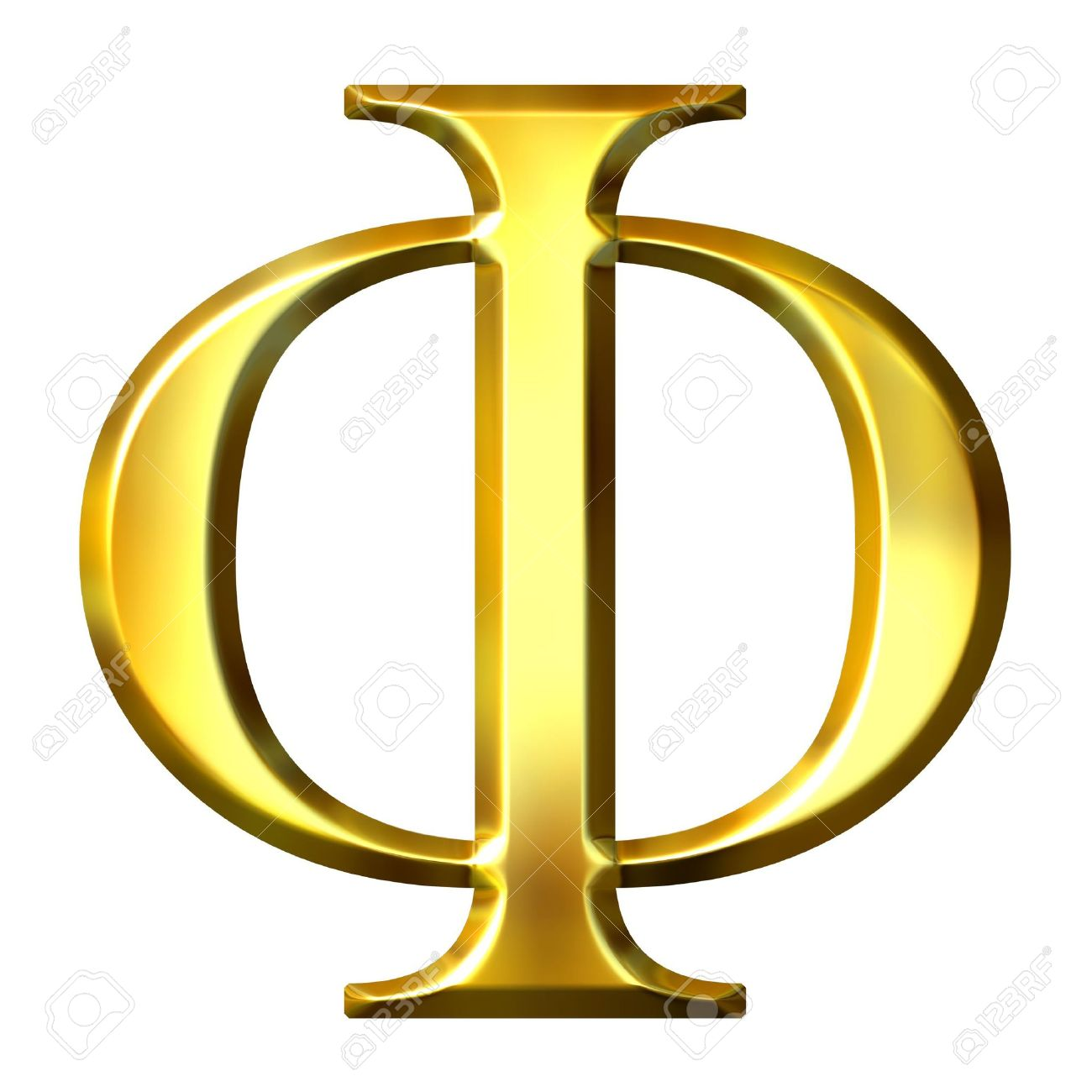# Why Phi Mantra?

“Phi” is the 21st letter of Greek alphabets. It is used to represent the “Golden ratio”, the magical ratio which can be find everywhere in the nature. It appears in most patterns of the nature from floral patterns to Galaxies, exploring it could help us to understand the secrets of this universe. Phi is the symbol for Fibonacci number series. Invented by Indian mathematician Virahanka and developed by western mathematician Fibonacci.
Fibonacci numbers are the most importance numbers in predicting the future. Magi society an astrological society claims that the planetary motion ratio of all the planets in the solar system comes in Fibonacci ratios. i.e the ratio between the time taken to a planet to finish its journey around the sun to its next planet’s time taken for the same is a Fibonacci ratio.phi mantra
In financial markets it is known about the importance of Fibonacci support levels. In a trending market the supports and resistances are usually Fibonacci numbers. So the purpose of this website is to understanding the magic of “PHI” that is why it is Phi mantra.